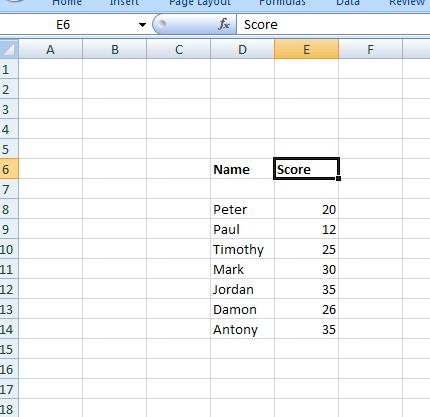### Ranking Values

Imagine that we have the following data:And we want to find the people with the highest 3 marks. Note for this example, we will assume that there are no two people with exactly the same score.

The function RANK allows us to do this. It needs 2 arguments – the cell that we are comparing and the range over which we are comparing:

RANK(A7, A2:A20)

Will give us the ranking of Cell A7 in the range A2:A20. So if we apply to this to our formulae and copy down:We see that the highest marks are Anthony, Mark and then Jordan – receiving 35, 30 and 28 marks respectively.

Note so far we have only determined the ranking of the names – not the actual names of the people. To do that we need to find the position of the ranking – i.e the fact that the 2nd largest value is on the 4th row of our data, and that the 4th largest is on the 6th row. This is achieved by doing the MATCH function:

MATCH(A2, A2:A10,0)

Will give us the position of contents of the cell A2 In the range A2:A10. So we can add another column to determine the position of the nth largest item:And then we can set space on the workbook to display the three largest values:And we state the position of the 3 largest items – using the MATCH function that we have just defined:This will return the position in the list of the largest 3 items. We can then use in the argument of an INDEX function to return the name of the students with the 3 highest marks: